Cruise down to question 4 for a question about traveling!

# Direct and Inverse Variation

This Math quiz is called 'Direct and Inverse Variation' and it has been written by teachers to help you if you are studying the subject at middle school. Playing educational quizzes is a fabulous way to learn if you are in the 6th, 7th or 8th grade - aged 11 to 14.

It costs only \$12.50 per month to play this quiz and over 3,500 others that help you with your school work. You can subscribe on the page at Join Us

What happens to the area of a square if you double the length of each side? Or the volume of a balloon, if the radius doubles? Questions like this can be modeled using variation – how does one variable change in relation to another one? There is a standard approach to solving these types of problems, see how good you are in this middle school Math quiz on direct and inverse variation.

We can recognize questions on variation by some of the words and phrases used – ‘directly proportional’ indicates that one variable increases when the other one also increases. If you see ‘inversely proportional’ then this means one variable decreases as the other one increases. As soon as you see this in a question you can write down a statement using the proportionality symbol, ∝.

The next stage is to introduce the constant of proportionality, usually the letter k, and turn this into an equation. At this point if you have been given numerical data, you can substitute it into the equation, to find a value for k.

It is also a good idea to know what shape graph each type of variation would have. In direct proportion problems, the curve or line always goes through the origin. Inverse proportion problems have a reciprocal graph, to indicate the decrease of one variable.

Question 1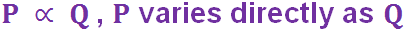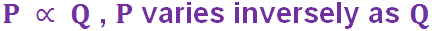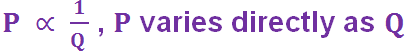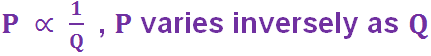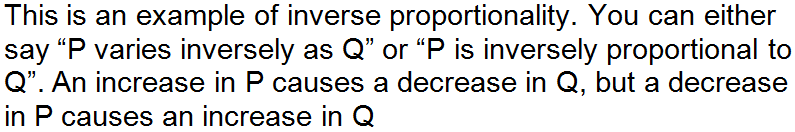Question 2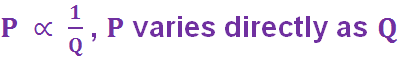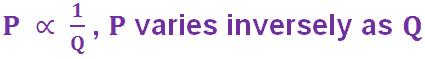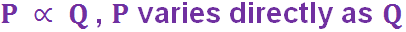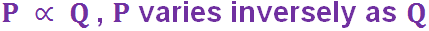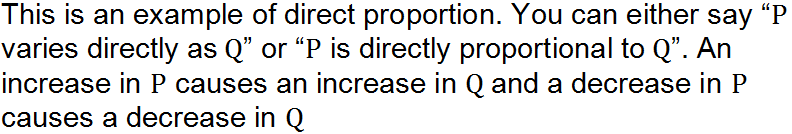Question 3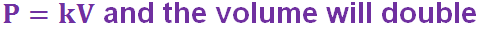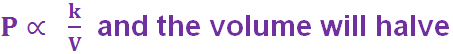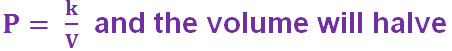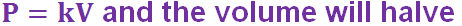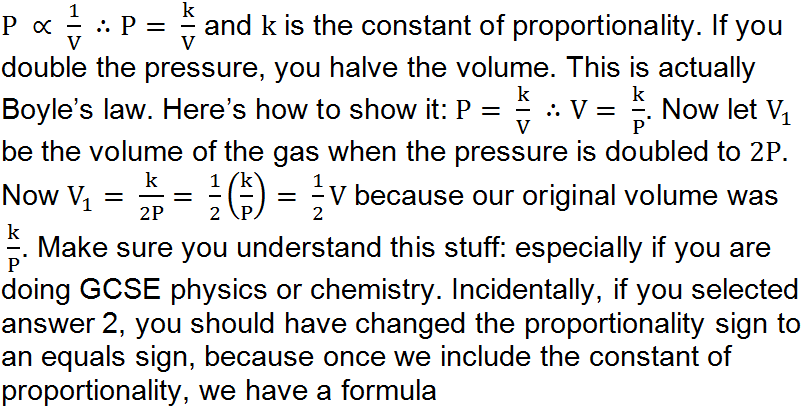Question 4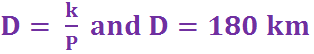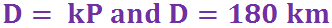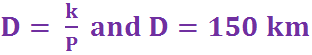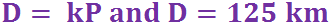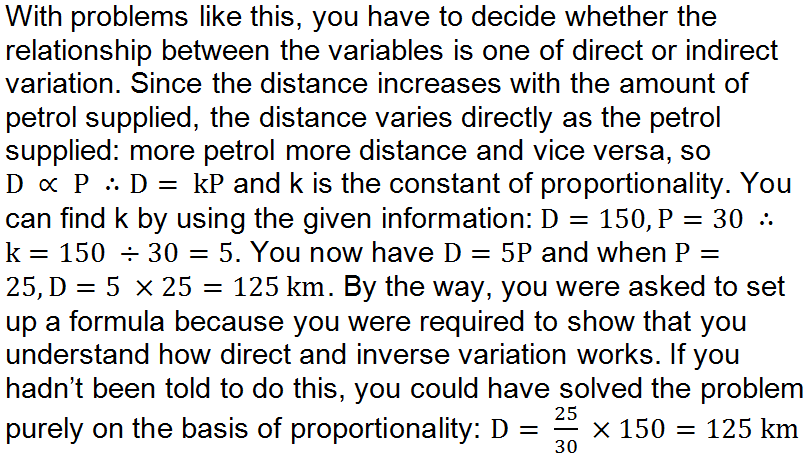Question 5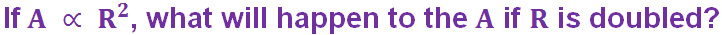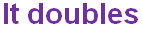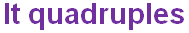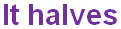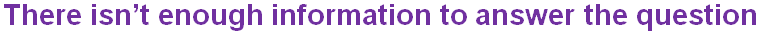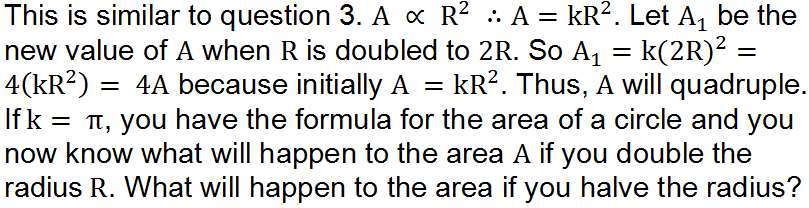Question 6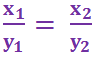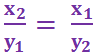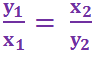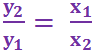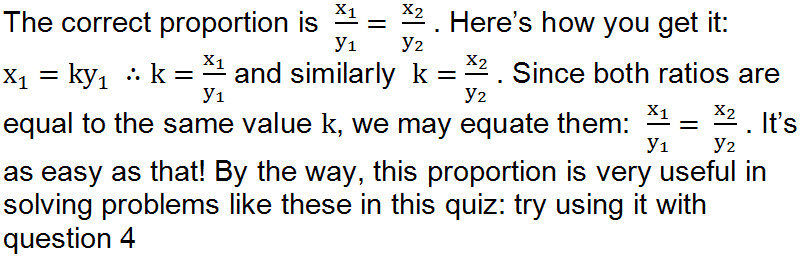Question 7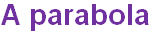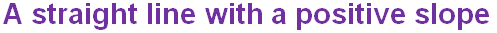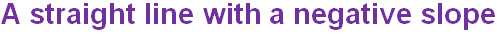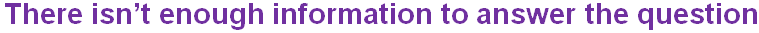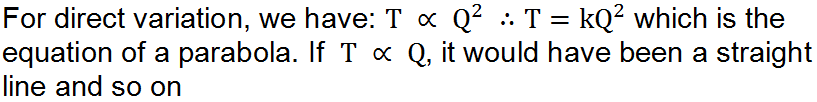Question 8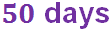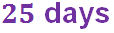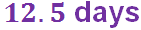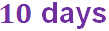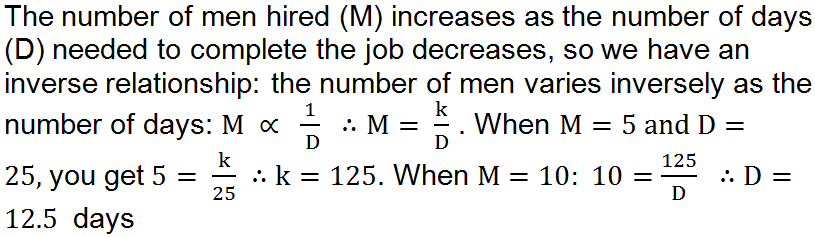Question 9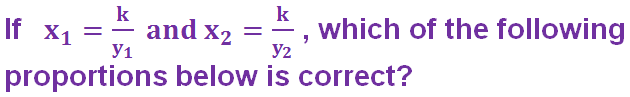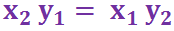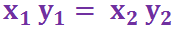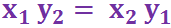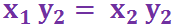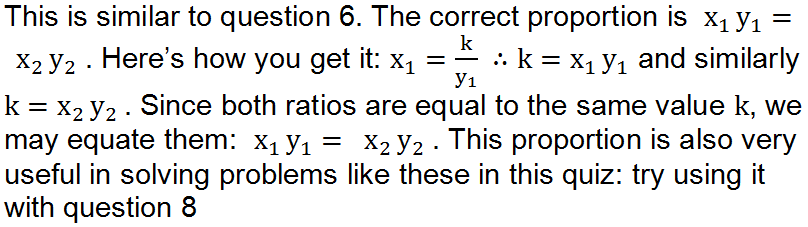Question 10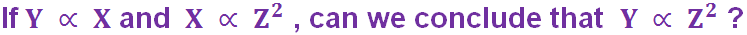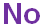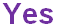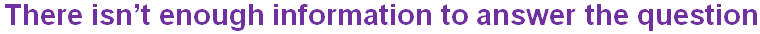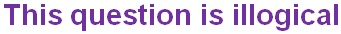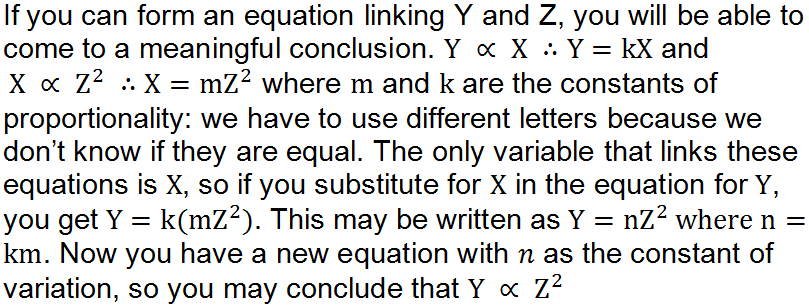Author:  Frank Evans